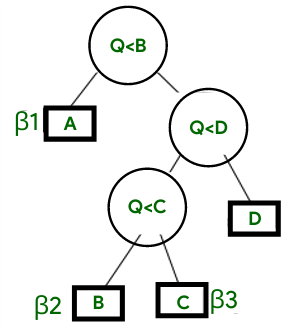Related Articles

# Introduction of Hu-Tucker algorithm

• Last Updated : 29 Jun, 2021

Introduction of the Hu-Tucker algorithm :
The Hu-Tucker algorithm helps to compress some order of blocks, assuming that you have a certain natural order for the following, then if the strings and the notes are taken into account, then taking them into account, they are sorted in numbers.
The question now arises about how we can build the order so that the compression fits perfectly, and still pose the same code without altering the nature of the strings, i.e. the order of them.
Eg – “Geeks for Geeks” remains “Geeks for Geeks” not “for Geeks Geeks“.

Hence, we need to conserve order too while compressing things, hence sorting strings as number is the way to go.
Well, to address all these issues, we have a simple algorithm called Hu-Tuker that helps to achieve accurate results even though being pretty old.

Understanding the Hu-Tucker :
This algorithm is classified into 3 different phases and the time complexity of each of the methods is then alternatively assessed. After that, the trials are taken and then justified for the amount which is charged for the complexity. Out of all the 3 phases, there are 2 independent methods, which take O(n2) and O(nlogn) complexity respectively.
Thus, the dividing property or the distinguishing line, as some might say, would be the abstract relationship derived from them.
And then, after numbering, the decoding and the encoding process is taken care of by the algorithm itself, just after keying in the values!

The Hu–Tucker Code is the alphabetical search tree’s binary code.

Let’s look at one tree to learn it better :The hu-tucker tree.

As in the above figure, pass  1, we see from the trees, that certain strings (classified as Beta) store certain values with fixed length, something which we addressed above. Now, after running these transformations overtime, they get weak for the algorithm and thus get compressed eventually. Also, as we added the numbers, we can rest-assured that the order would be perfect!

After that comes pass 2, which takes the most commonly appearing characters and groups them accordingly, then compresses them and then decompresses them, so that the storage space utilized is less, as the frequent characters are now grouped!

Although this algorithm is quite old for working with, but the classical essence of compression is said to be derived from this. Moreover, there are still certain advantages of using this method over the other as the latency and compression drops in this method are really less. We will now see a short and crisp example of the Hu-Tucker algorithm to better understand the learning curve using certain nodes and alphabet symbols.

The Algorithm for implementing Hu Tucker follows below :

```1. 'terminal' label node 0, ... n-1
2. Repeat repetitions (n - 1):
(a) find a pair I j) to be I I < j;
(ii) node I or j is not labelled "none" and
(iii) no node (i+1, etc.)
(iv) weight[i] + weight[j] is minimal,
(v) I is not unique after (iv) and (v)
3. j is minimal if not unique following the selection process.
(a) Mix node j and
(b)save node j as new node I
(c) Weight[i]+= Weight[j] Weight[i] Weight
(d) Node I 'interior'
(e) Node I 'not' Label node```

Applications of Hu-Tucker :
Let us look at 2 applications of the Hu Tucker Algorithm for better understanding.

1. Implementing Search :
As Hu Tucker is a compression and pattern finding algorithm, it can be used for searching patterns in databases as it uses tree structure and all the searching techniques use binary search as extensively as possible.
Example:
Let us denote the number of edges of the trees as ‘i’, which is a subset of another set of elements such that  i ∈ {1, 2, . . . , n}, then using the Hu-Tucker algorithm, we can find the weight in the given set and can yield the search result.
2. Minimizing Cost Functions :
Trailing from above, the Hu-Tucker algorithm is also a compression technique and thus, helps find out the minimum cost function calculations.

The formula used for minimizing is:

```La(w,l) , loga∑
Xn
i=1
w(i)a
l(i)```

Drawbacks of Hu-Tucker :
Despite the fact that the Hu-Tucker algorithm is a neat and profound algorithm, still like with all other algorithms, it has drawbacks and places where it fails. The algorithm is somewhat strange as it depends upon a lot of other information which sometimes is not provided. Like in Application 2 above, the logarithmic function requires too much data, which is normally not a requirement with modern compression algorithms. Also, as this algorithm has aged, newer ones pose a better and less time complexity as compared to this, hence it feels a little outdated and inferior to them!

Conclusion :
This was all about a short introduction to the Hu-Tuckker algorithm. Hope this article helped you with grasping the brief intro to it and helps you in paving your way forward!

Attention reader! Don’t stop learning now. Get hold of all the important DSA concepts with the DSA Self Paced Course at a student-friendly price and become industry ready.  To complete your preparation from learning a language to DS Algo and many more,  please refer Complete Interview Preparation Course.

In case you wish to attend live classes with experts, please refer DSA Live Classes for Working Professionals and Competitive Programming Live for Students.

My Personal Notes arrow_drop_up